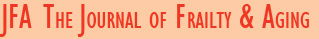AND optionOR option

# Archives

Back to all journals

### journal articles

AN ULTRASOUND PREDICTION EQUATION TO ESTIMATE DXA-DERIVED BODY FATNESS FOR MIDDLE-AGED AND OLDER CAUCASIAN ADULTS

T. Abe, J.P. Loenneke, R.S. Thiebaud

J Frailty Aging 2019;8(2):79-84

Background & Objectives: Currently, only one study has used dual-energy X-ray absorptiometry (DXA)-derived percent body fat (BF%) as the criterion measure to develop ultrasound prediction equations to estimate BF% in adults between the ages of 50 and 80 years. The aim of this study was to examine the relationship between BF% estimated from subcutaneous fat thickness using a previously published Japanese-based prediction equation and DXA-derived BF% in Caucasian middle-aged and older adults. A secondary aim was to develop a new prediction equation for Caucasian adults if the previously published equation did not predict BF% well in Caucasians. Design: Cross-sectional study. Participants & Measurements: One-hundred and two Caucasian adults aged 50-76 years (59 men and 43 women) had ultrasound fat thickness and DXA values measured. A new BF% prediction model was developed using ordinary least squares multiple linear regression. Results: There was a strong correlation between ultrasound predicted and DXA-derived BF% (r = 0.882, p<0.001). Bland-Altman analysis did not indicate a bias in the prediction of BF% for Caucasian adults (r = -0.092, p>0.05). However, the predicted BF% was significantly higher compared to DXA-derived BF% (approximately 4%). A newly developed nonlinear prediction model used to estimate BF% was significant [F(17,84) = 33.44, p<0.001] with an R2 of 0.871 and an adjusted R2 of 0.845. When examining the stability of the model, bootstrapping (n=1000) resulted in an optimism value of 0.1135 so that the corrected R2 was 0.758. After removing an outlier, the model was significant [F(17,83) = 34.82, p<0.001] and it’s R2 was 0.877 and adjusted R2 was 0.852. Conclusion: The developed equation was stable with a high degree of variance compared to results from previous studies. The results of this study also suggest that ethnicity should be considered when choosing which prediction equations should be used to estimate BF%.

CITATION:
T. Abe ; J.P. Loenneke ; R.S. Thiebaud (2019): An ultrasound prediction equation to estimate DXA-derived body fatness for middle-aged and older Caucasian adults. The Journal of Frailty and Aging (JFA). http://dx.doi.org/10.14283/jfa.2019.8